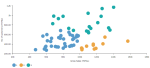# MLLIB.CLUSTER(imputer, n_clusters, n_iter, columns)

K-means is one of the most commonly used clustering algorithms that clusters the data points into a predefined number of clusters. It includes a paralleled variant of the k-means++ for clusters initialization K-means method.

###### Parameters
• imputer – Strategy for dealing with null values:

• 0 – Replace null values with ‘0'

• 1 – Assign null values to a designated ‘-1' cluster

• number_of_clusters – Number of clusters which the algorithm should find, integer.

• number_of_iterations – Maximum number of iterations (recalculations of centroids) in a single run, integer.

• columns – Dataset columns or custom calculations.

Example: MLLIB.CLUSTER(0, 3, 20, sum([Gross Sales]), sum([No of customers])) used as a calculation for the Color field of the Scatterplot visualization.###### Input data
• Size of input data is not limited.
• Without missing values.
• Character variables are transformed to numeric with label encoding.
###### Result
• Column of integer values starting with 0, where each number corresponds to a cluster assigned to each record (row) by the K-means algorithm.
###### Key usage points
• Fast and computationally efficient, very high scalability.
• Practically works well, even if some of its assumptions are broken.
• General-purpose clustering algorithm.
• When the approximate number of clusters is known.
• When there is a low number of outliers.
• When the clusters are spherical, with approximately same number of observations, density and variance.
• Euclidean distances tend to be more inflated with higher number of variables (curse of dimensionality).
• By calculating Euclidean distance, the algorithm makes assumption of only numeric input variables. One-hot encoding of categorical variables is a workaround suitable for a relatively low number of categories to encode.
• K-means makes an assumption that we deal with spherical clusters and that each cluster has roughly equal numbers of observations, density and variance, otherwise the results might be misleading.
• It always finds clusters in the data, even if no natural clusters are present.
• All data points are assigned to a cluster, even though some of them might be just random noise.
• Sensitivity to outliers.
###### Example

For the whole list of algorithms, see Data science built-in algorithms.